Geometry

# Triangles - Identify Similar Triangles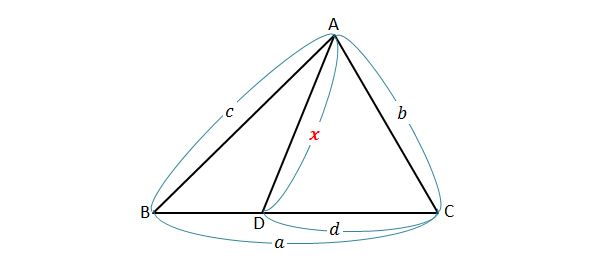In the above diagram, if $a=18,$ $b=12,$ $c=14$ and $d=8,$ what is the measure of $\overline{AD} ?$

Note: The above diagram is not drawn to scale.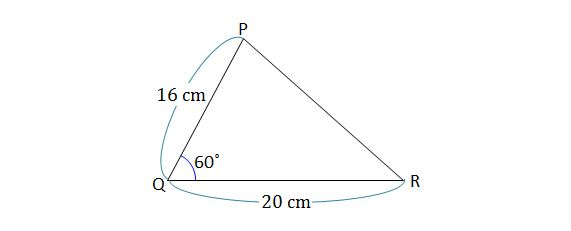Given $\triangle PQR$ with side lengths $\lvert{\overline{PQ}}\rvert=16\text{ cm},$ $\lvert{\overline{QR}}\rvert=20\text{ cm}$ and $\angle PQR=60^\circ,$ which of the following triangles is similar to $\triangle PQR ?$

(a) $\triangle ABC$ with side lengths $\lvert{\overline{AB}}\rvert={15}\text{ cm},$ $\lvert{\overline{AC}}\rvert={12}\text{ cm}$ and $\angle BAC=60^\circ$
(b) $\triangle DEF$ with side lengths $\lvert{\overline{DE}}\rvert={8}\text{ cm},$ $\lvert{\overline{DF}}\rvert={10}\text{ cm}$ and $\angle EDF=80^\circ$
(c) $\triangle GHI$ with angles $\angle GHI=60^\circ$ and $\angle GIH=80^\circ$
(d) $\triangle JKL$ with side lengths $\lvert{\overline{JK}}\rvert={20}\text{ cm},$ $\lvert{\overline{KL}}\rvert={18}\text{ cm}$ and $\lvert{\overline{JL}}\rvert={16}\text{ cm}$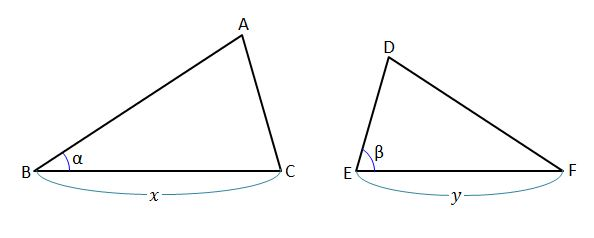In the above diagram, $x=21,$ $y=14,$ $\alpha={35}^\circ,$ and $\beta={75}^\circ.$ Which condition would mean that the two triangles are similar?

Note: The above diagram is not drawn to scale.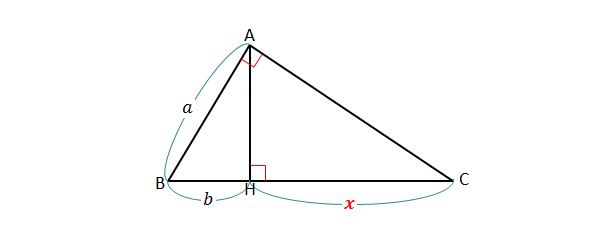In the above diagram, if $a=30,$ $b=25$ and $\angle BAC=\angle AHC=90^\circ,$ what is the measure of $\overline{HC} ?$

Note: The above diagram is not drawn to scale.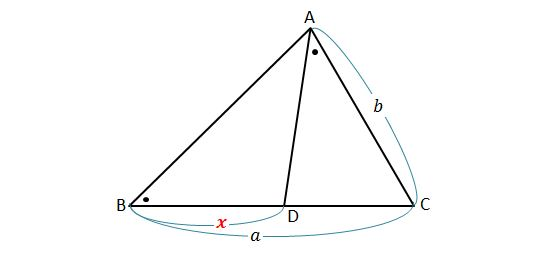In the above diagram, if $a=45,$ $b=30$ and $\angle ABC=\angle DAC,$ what is the measure of $\overline{BD} ?$

Note: The above diagram is not drawn to scale.

×